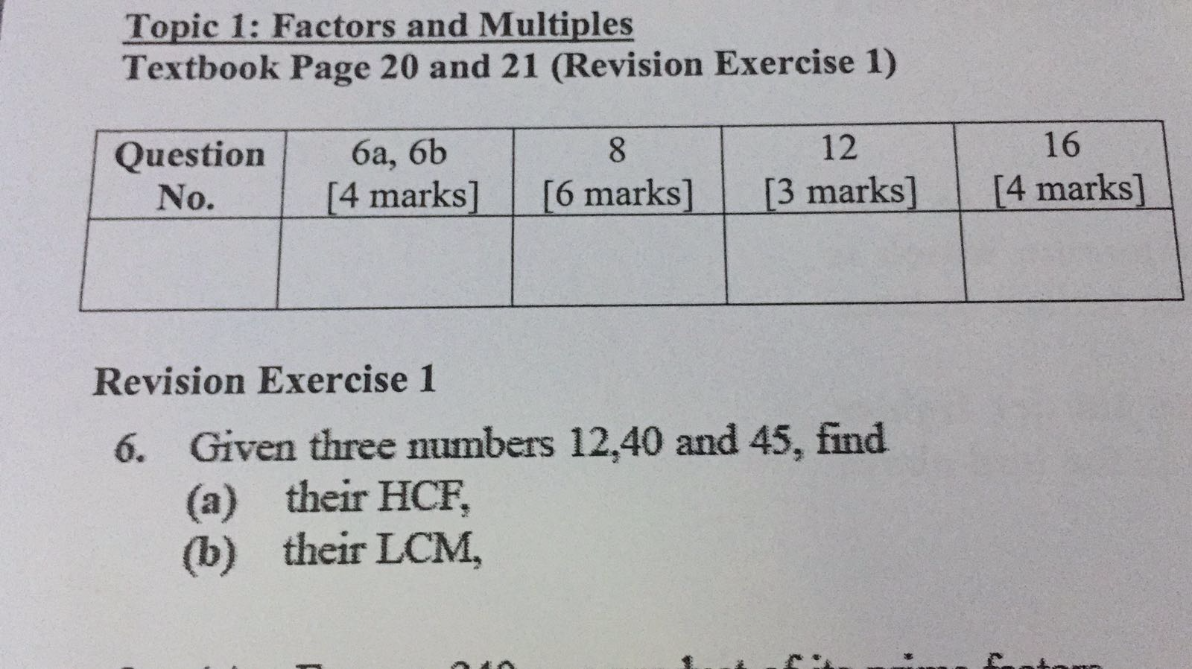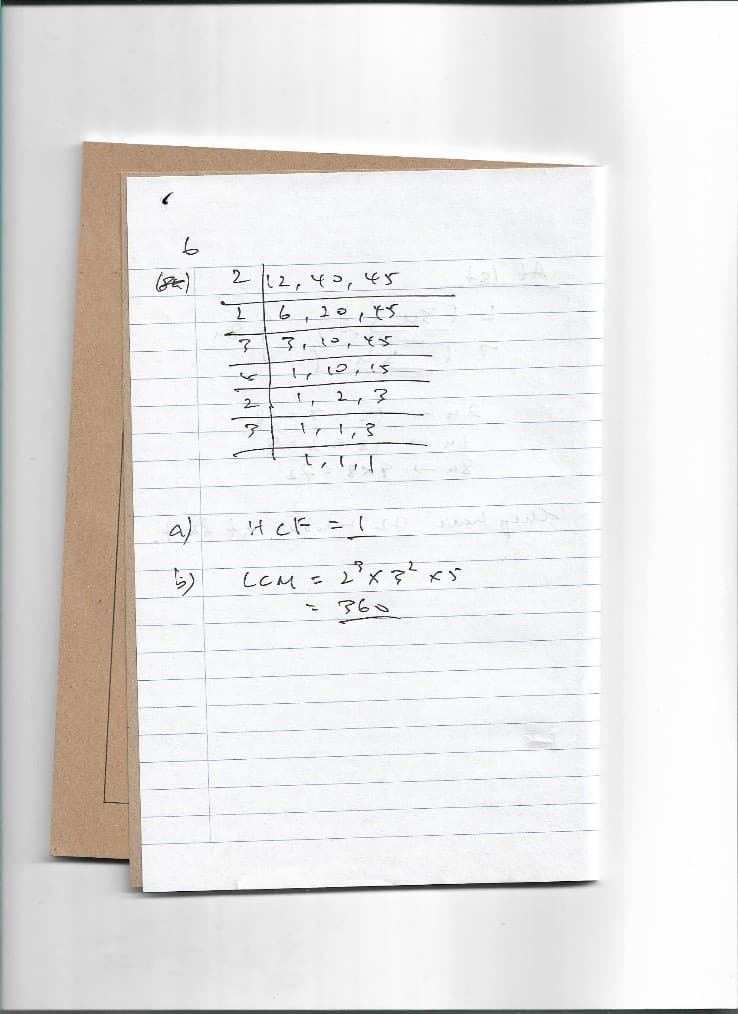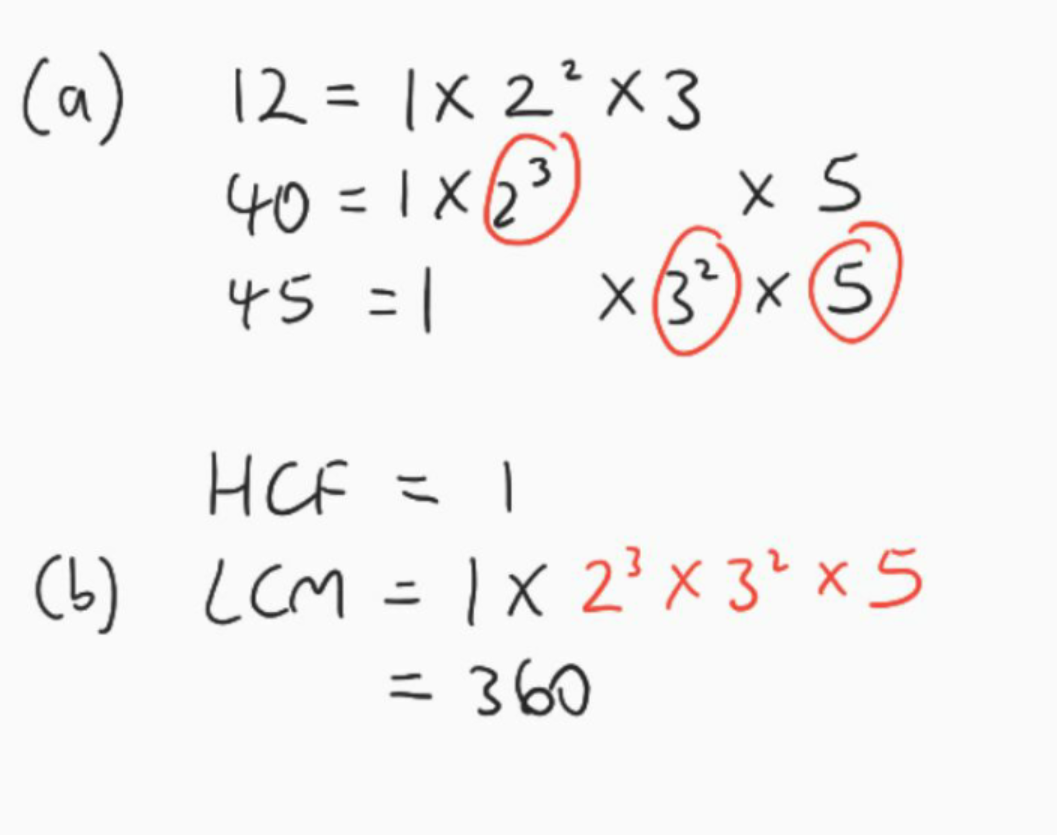# QuestionHow do i solve?

Source: temasek secondary

12 = 2^2 x 3 x 1

40 = 2^3 x 5 x 1

45 = 3^2 x 5 x 1

HCF = 1 (all numbers have a factor of 1)

LCM = 2^3 x 3^2 x 5

= 360

0 Replies 0 Likes

12 = 2 x 2 x 3
40 = 2 x 2 x 2 x 5
45 = 3 x 3 x 5

HCF = 1
LCM = 2^3 x 3^2 x 5 = 360

Ans : HCF = 1 and LCM = 360.

0 Replies 0 Likes

HCF: 12=1x2x2x3
40=1x2x2x2x5

45=1x3x3x5

HCF=3

LCM= 1X2X2X2X3X3X5=360

0 Replies 0 Likes0 Replies 0 Likes0 Replies 0 Likes